# GMAT Math : DSQ: Understanding powers and roots

## Example Questions

← Previous 1 2 Next →

### Example Question #11 : Dsq: Understanding Powers And Rootsis a positive real number. True or false:is a rational number.

Statement 1: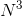is a rational number.

Statement 2: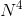is a rational number.

Possible Answers:

EITHER statement ALONE is sufficient to answer the question.

BOTH statements TOGETHER are insufficient to answer the question.

Statement 2 ALONE is sufficient to answer the question, but Statement 1 ALONE is NOT sufficient to answer the question.

Statement 1 ALONE is sufficient to answer the question, but Statement 2 ALONE is NOT sufficient to answer the question.

BOTH statements TOGETHER are sufficient to answer the question, but NEITHER statement ALONE is sufficient to answer the question.

Correct answer:

BOTH statements TOGETHER are sufficient to answer the question, but NEITHER statement ALONE is sufficient to answer the question.

Explanation:

Statement 1 alone is not enough to determine whetheris rational or not;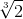andboth have rational cubes, but onlyis rational. By a similar argument, Statement 2 alone is insufficient.

Assume both statements are true.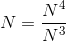, the quotient of two rational numbers, which must itself be rational.

### Example Question #11 : Powers & Roots Of Numbers

Let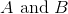be positive integers. Is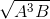an integer?

Statement 1:is a perfect square.

Statement 2:is an even integer.

Possible Answers:

Statement 1 ALONE is sufficient to answer the question, but Statement 2 ALONE is NOT sufficient to answer the question.

Statement 2 ALONE is sufficient to answer the question, but Statement 1 ALONE is NOT sufficient to answer the question.

EITHER statement ALONE is sufficient to answer the question.

BOTH statements TOGETHER are sufficient to answer the question, but NEITHER statement ALONE is sufficient to answer the question.

BOTH statements TOGETHER are insufficient to answer the question.

Correct answer:

BOTH statements TOGETHER are insufficient to answer the question.

Explanation:

We examine two examples of situations in which both statements hold.

Example 1: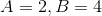Then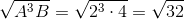32 is not a perfect square, sois not an integer.

Example 2: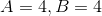Then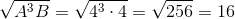, makingan integer.

In both cases, both statements hold, but in only one,is an integer. This makes the two statements together insufficient.

### Example Question #11 : Dsq: Understanding Powers And Roots

Simplify: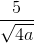Possible Answers: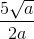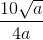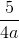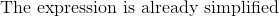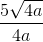Correct answer:Explanation:

When we are faced with a radical in the denominator of a fraction, the first step is to multiply the top and bottom of the fraction by the numerator: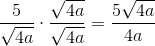We can then reduce the fraction to: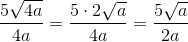### Example Question #311 : Arithmeticandare integers. Is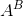positive, negative, or zero?

Statement 1:is negative.

Statement 2:is odd.

Possible Answers:

Statement 2 ALONE is sufficient to answer the question, but Statement 1 ALONE is not sufficient to answer the question.

Statement 1 ALONE is sufficient to answer the question, but Statement 2 ALONE is not sufficient to answer the question.

Both statements TOGETHER are insufficient to answer the question.

Both statements TOGETHER are sufficient to answer the question, but neither statement ALONE is sufficient to answer the question.

Either statement ALONE is sufficient to answer the question.

Correct answer:

Both statements TOGETHER are sufficient to answer the question, but neither statement ALONE is sufficient to answer the question.

Explanation:

A negative integer to an even power is positive:

Example: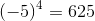A negative integer to an odd power is negative:

Example: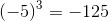A positive integer to an odd power is positive:

Example: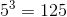So, as seen in the first two statements, knowing only that baseis negative is insufficient to detemine the sign of; as seen in the last two statements, knowing only that exponentis odd is also insufficient. But by the middle statement, knowing both facts tells usis negative.

The answer is that both statements together are sufficient to answer the question, but neither statement alone is sufficient.

← Previous 1 2 Next →

### All GMAT Math Resources